| my account | login-logout | resources | support | catalog | home | get webcard |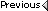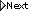» Online Classroom   » Inland and Coastal Navigation   » Public Discussion of Inland and Coastal Navigation   » Problem 2-5, B,C and D

Author Topic: Problem 2-5, B,C and D
 James Muniz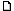posted January 30, 2005 08:32 PM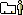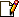How did you come up with the interpolated deviations for B, C, and D. I tried using the tables provided and what I filled in but cannot just get it.1 330 1.0w 330 1.02 345 4.8e Des Heading 340 ? 30 5.8 105.8 devided by 30 = .1933333 = .2 X 10 = * 2, 2 + 1 = 3 340 + 5 var = 345 T - 3 =342 *(I added because to get from 1.w to 4.8e I'll have to add)problem (B) was ok but c and d c) 1 030 16.9 E 030 16.9 E 2 045 20.0 E Des Heading 033 ? 15 3.1 33.1 devided by 15 = .2066666 X 3 = .6199998 = .6216.9 + .62 = 17.5, 033 T + 5 var = 038 - 17.5 = 20.5 but the deviation given in the answers is 15.2---what am I doing wrong.
 David Burchposted January 31, 2005 06:00 PMHave you seen the article in the notes call Robert's method of interpolation? It is on the last page of the Chart Problems book. Also it is in the Resources somewhere, but i cannot find it for the moment. It shows a straight forward method with several examples.Please see if that helps and let me know.===in the meantime, it seems the main question is (for example) where does the dev = 3.5°E come from for problem B, since all the rest is given in the answers.the original question is when variation is 5° W what compass course do you steer to make good a true course of 340 T, taking into account a given deviation table.The simplest approach is to use the table (TV Makes Dull Companions) Add east going up, which is called the correting direction, which is the same as subtracting east going down or the same as adding west going down (see course book notes)T = 340 = givenV = 5 W = given M = (345 M ) we are going down so we addD = must figure dev from tableC = 345 + dev if W or 245 - dev if E.so what is the dev at 345 M.From the given dev table we know:at 315C dev = 6.8 Wandat 000C dev = 10.5 Ethe problem is first, the table is not in very small steps, ideally we would want this every degree, but compass adjusters tend to give it just at the cardinal and intercardinal points, and second, on top of that, we have W on one and then E on the next. So we have to interpolate carefully.from 315 to 000 is 45° of compass heading and from dev 6.8 W to 10.5 E is a total change in deviation of 6.8+10.5 = 17.3° (note we add them to find the "difference", since one is E and the other W). This is a rate of change of 17.3/45 = 0.38° of dev for each degree of compass heading.But we want the dev at 345 M, so we better start thinking in terms of magnetic. 315 C = 315C - 6.8W = 308.2 M and 000C = 000C + 10.5E = 010.5M. For comparison, lets now figure the dev rate in terms of magnetic degrees. we still have a dev difference of 17.3°, but now the change in magnetic heading is 308.2 to 010.5, which is 360-308.2 = 51.8, and 51.8+ 10.5 = 62.3°. The rate of change of the dev in this heading range when expressed in terms of magnetic degrees is 17.3/62.3 = 0.28° per degree of magnetic heading.We want the dev at 345M. We know it at 308.2 M, which is 36.8° lower.... hence we can take the value at 308.2M and correct it to 345M since we know the rate it is changing (0.28° per degree ). the change is 36.8x0.28 = 10.3°.at 308.2M the dev is 6.8W and it is going down to 10.5E at 010.5, so we have 6.8 W - 10.3° = 3.5E, which is the answer listed in the Chart Problems.Hopefully this solution will help resolve the other two as well. --david From: Starpath, Seattle, WA
 James Munizposted February 01, 2005 09:47 AMThat clarified alot and I see what I needed to do, not so confused in Colorado.

 All times are Pacific